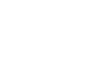Which theory was given by Einstein? - letsdiskussVanisha Anand

Student | Posted | Science-Technology

# Which theory was given by Einstein?

1
0

student | Posted

Albert Einstein was an German scientist and a famous physicists. He has given several important inventions and concepts to the world. Some of the theories are Theory of Relativity, Special relativity, general relativity, photoelectric effect, energy- mass equivalence, Theory of Brownian notion, gravitational wave, cosmological constant, Plank- Einstein equivalence and many more. He was awarded with Nobel prize in physics, Barnard medal, Gold medal of the royal, Time person of the country, Copley medal and many more.1
0

| Posted

Albert Einstein proposed a theory of special relativity in 1905. One of the important theory ever published in physics. This theory explain that how speed affects mass, time and space. This theory is defined by the equation E = mc^2. This theory is applied to special cases.

General relativity is the theory of gravitation developed by Albert Einstein in 1907.0
0